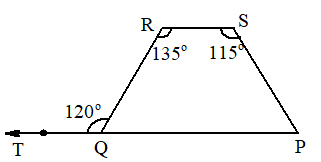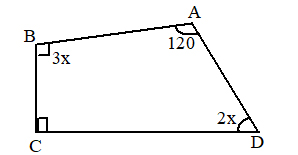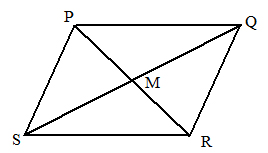# SSC Worksheet for Chapter - 2 Quadrilaterals Class 8

## Find SSC Worksheet for chapter-2 Quadrilaterals class 8

CLASS-8

BOARD: SSC

Mathematic Worksheet - 2

For otherSSC Worksheet for class 8 Mathematiccheck out main page of Physics Wallah.

1. Three angles of a quadrilateral are 130°,90°,60°. Then the fourth angle is
1. 50°
2. 60°
3. 70°
4. 80°
2. In a quadrilateral∠A :∠B :∠C ∠ D:: 5:9:16:10. Then∠B=
1. 45
2. 81
3. 105
4. 80
3. The∠Bangle in the figure is1. 70°
2. 60°
3. 65°
4. 45°
4. The angle∠ Bin the figure is1. 30°
2. 60°
3. 90°
4. 120°
5. If the length of diagonal of a square is 13 units then the length of its side is
1. 13√2/2
2. 12√3/5
3. 3√13/2
4. 8√2/7
6. In the figureQMR =90°, MR = 5 and SM = 7, then1. √49
2. √54
3. √74
4. √84
7. In a parallelogram PQRS,=2x,Q = 3x+30. ThenR =
1. 50°
2. 60°
3. 120°
4. 80°
8. Prove that, two opposite vertices if a parallelogram are equidistant from the diagonal not containing these vertices.
9. The ratio of two sides of parallelogram is 3:4. If its perimeter is 112cm, find the length of all the sides of that parallelogram.
10. Prove that: Base angles of an isosceles trapezium are congruent.
11. Prove that in a rhombus with angle of 60°, the shorter diagonal divides the rhombus into two equilateral triangles.
12. The diagonals of rectangle PQRS intersect at M. IfQMR = 50°findMPS.